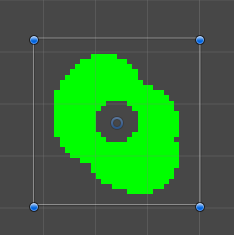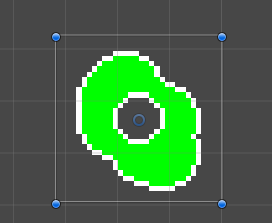# Shaders - offset texture coordinates by a single pixel

I’m trying to make an outline shader for sprites, so I need to check adjacent pixels in the texture. However, the tex2d() shader function expects 0…1 for x/y coordinates, so if I want to offset by a single pixel, I would have to know the texture’s size. Since I use unity’s sprite packer, these are usually fairly large sprites.

Is there a way to check a neighboring pixel in the texture? Is there a way to get the texture size in pixels, so I can calculate this myself?

I adapted the default sprite shader to do roughly what you want:

``````Shader "Custom/OutlinedSprite"
{
Properties
{
[PerRendererData] _MainTex ("Sprite Texture", 2D) = "white" {}
_Color ("Tint", Color) = (1,1,1,1)
_OutlineColor ("Outline", Color) = (1,1,1,1)
[MaterialToggle] PixelSnap ("Pixel snap", Float) = 0
}

{
Tags
{
"Queue"="Transparent"
"IgnoreProjector"="True"
"RenderType"="Transparent"
"PreviewType"="Plane"
"CanUseSpriteAtlas"="True"
}

Cull Off
Lighting Off
ZWrite Off
Blend One OneMinusSrcAlpha

Pass
{
CGPROGRAM
#pragma vertex vert
#pragma fragment frag
#pragma multi_compile _ PIXELSNAP_ON
#include "UnityCG.cginc"

struct appdata_t
{
float4 vertex   : POSITION;
float4 color    : COLOR;
float2 texcoord : TEXCOORD0;
};

struct v2f
{
float4 vertex   : SV_POSITION;
fixed4 color    : COLOR;
half2 texcoord  : TEXCOORD0;
};

fixed4 _Color;
fixed4 _OutlineColor;
float _TexWidth;
float _TexHeight;

v2f vert(appdata_t IN)
{
v2f OUT;
OUT.vertex = mul(UNITY_MATRIX_MVP, IN.vertex);
OUT.texcoord = IN.texcoord;
OUT.color = IN.color * _Color;
#ifdef PIXELSNAP_ON
OUT.vertex = UnityPixelSnap (OUT.vertex);
#endif

return OUT;
}

sampler2D _MainTex;
float4 _MainTex_TexelSize;

fixed4 frag(v2f IN) : SV_Target
{
fixed4 c = tex2D(_MainTex, IN.texcoord) * IN.color;

if (c.a == 0) {
return fixed4(0, 0, 0, 0); // Skip outline for transparent pixels
}

// Get the colors of the surrounding pixels
fixed4 up = tex2D(_MainTex, IN.texcoord + fixed2(0, _MainTex_TexelSize.y));
fixed4 down = tex2D(_MainTex, IN.texcoord - fixed2(0, _MainTex_TexelSize.y));
fixed4 left = tex2D(_MainTex, IN.texcoord - fixed2(_MainTex_TexelSize.x, 0));
fixed4 right = tex2D(_MainTex, IN.texcoord + fixed2(_MainTex_TexelSize.x, 0));

// This method uses an if statement
if (up.a * down.a * left.a * right.a == 0) {
c.rgb = _OutlineColor.rgb;
}

/* This method doesn't use an if statement, but it won't work for sprites with semi-transparency.

I prefer the previous method because I don't notice any performace difference between the two.

float isNotOutline = up.a * down.a * left.a * right.a;
c.rgb = isNotOutline * c.rgb + (1-isNotOutline) * _OutlineColor;

*/

c.rgb *= c.a;
return c;
}
ENDCG
}
}
}
``````

The outline here will appear inside the sprite. It’s pretty easy to change so that the outline is on the outside of the sprite, but there are some edge cases that you have to handle when you’re at the edge of a sprite.

Here is the original sprite, rendered using a normal sprite renderer:Here is the sprite with the sprite renderer’s material set to one that uses the outline shader:Edit:
To answer your question, `float4 _MainTex_TexelSize;` gets a few numbers that are relevant to the texture called `_MainTex`.

It gives you a 4-vector where the first two elements are the size of one pixel in uv coordinates, and the last 2 elements is the resolution of the texture.

-how to offset by 1 pixel

I am not familiar with sprites, but I understand that you would like to have different uniform variables per different meshes. Ideally you would want to use 1 material to avoid any additional draw calls.

Whatever the case is, you are applying a texture on triangles of a mesh (quad etc).

A mesh is made of vertices which carry position, uv coordinates and a color.

You can color code the meshes’ vertices with color.
In shader, have several uniforms (20, 40, doesn’t matter, uniforms are cheap).
Test the for the color of vertex and use the appropriate offset uniform.
Say if you see that the vertex is yellow - apply the offset with offset = 0.2,
else if pinkish = 0.3 etc

.

That way you can tweak the values once, setting up all the uniforms via the c# script or directly in the editor via properties of material, ensuring that they look good on all the quads and save on the drawcalls, having just 1 material.

You will have to look into optimizing dynamic branching. Shaders don’t like loops and if/else statements. See how you can implement step / mix() functions